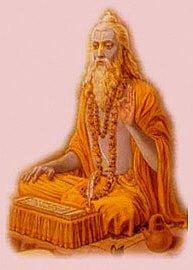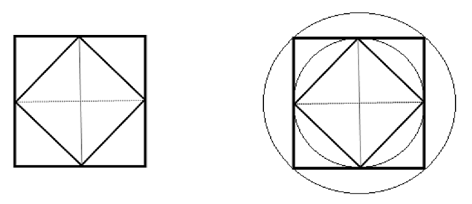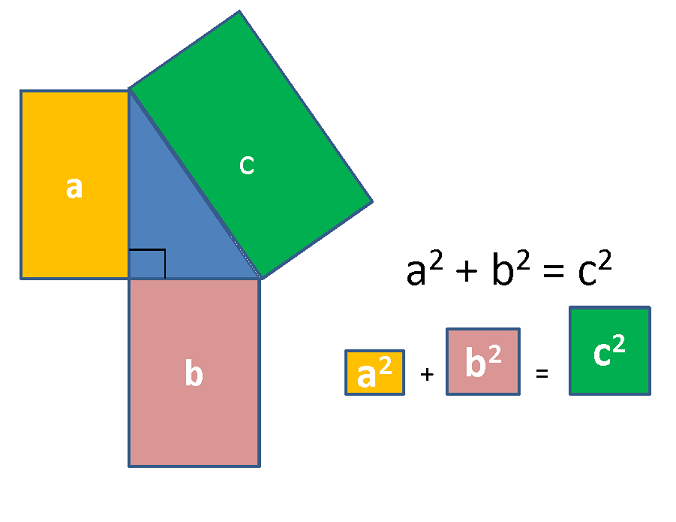Mathematics
Baudhayana | The Great Indian Mathematician
59.5k views

 1 Who is Baudhayana? 2 Works of Baudhayana 3 Baudhayana theorem 4 Summary 5 FAQs

21 September 2020

## Who is Baudhayana?Baudhayana (800 BC - 740 BC) is said to be the original Mathematician behind the Pythagoras theorem. Pythagoras theorem was indeed known much before Pythagoras, and it was Indians who discovered it at least 1000 years before Pythagoras was born! The credit for authoring the earliest Sulba Sutras goes to him.

It is widely believed that he was also a priest and an architect of very high standards. It is possible that Baudhayana’s interest in Mathematical calculations stemmed more from his work in religious matters than a keenness for mathematics as a subject itself. Undoubtedly he wrote the Sulbasutra to provide rules for religious rites, and it would appear almost certain that Baudhayana himself would be a Vedic priest.

The Sulbasutras is like a guide to the Vedas which formulate rules for constructing altars. In other words, they provide techniques to solve mathematical problems effortlessly.

If a ritual was to be successful, then the altar had to conform to very precise measurements. Therefore mathematical calculations needed to be precise with no room for error.
People made sacrifices to their gods for the fulfilment of their wishes. As these rituals were meant to please the Gods, it was imperative that everything had to be done with precision. It would not be incorrect to say that Baudhayana’s work on Mathematics was to ensure there would be no miscalculations in the religious rituals.

## Works of Baudhayana

Baudhayana is credited with significant contributions towards the advancements in mathematics. The most prominent among them are as follows:

### 1. Circling a square.

Baudhayana was able to construct a circle almost equal in area to a square and vice versa. These procedures are described in his sutras (I-58 and I-59).

Possibly in his quest to construct circular altars, he constructed two circles circumscribing the two squares shown below.Now, just as the areas of the squares, he realised that the inner circle should be exactly half of the bigger circle in area. He knew that the area of the circle is proportional to the square of its radius and the above construction proves the same. By the same logic, just as the perimeters of the two squares, the perimeter of the outer circle should also be $$\sqrt 2$$ times the perimeter of the inner circle. This proves the known fact that the perimeter of the circle is proportional to its radius. This led to an important observation by Baudhayana. That the areas and perimeters of many regular polygons, including the squares above, could be related to each other just as the case of circles.

### 2. Value of π

Baudhayana is considered among one of the first to discover the value of ‘pi’. There is a mention of this in his Sulbha sutras. According to his premise, the approximate value of pi is $$3.$$Several values of π occur in Baudhayana's Sulbasutra, since, when giving different constructions, Baudhayana used different approximations for constructing circular shapes.

Some of these values are very close to what is considered to be the value of pi today, which would not have impacted the construction of the altars. Aryabhatta, another great Indian mathematician, worked out the accurate value of $$π$$ to 3.1416. in 499AD.

### 3. The method of finding the square root of 2.

Baudhayana gives the length of the diagonal of a square in terms of its sides, which is equivalent to a formula for the square root of 2. The measure is to be increased by a third and by a fourth decreased by the 34th. That is it’s diagonal approximately. That is $$1.414216$$, which is correct to five decimals.

Baudhāyana (elaborated in Āpastamba Sulbasūtra i.6) gives the length of the diagonal of a square in terms of its sides, which is equivalent to a formula for the square root of 2:

samasya dvikaraṇī. pramāṇaṃ tṛtīyena vardhayettac caturthenātmacatustriṃśonena saviśeṣaḥ

Sama – Square; Dvikarani – Diagonal (dividing the square into two), or Root of Two

Pramanam – Unit measure; tṛtīyena vardhayet – increased by a third

Tat caturtena (vardhayet) – that itself increased by a fourth, Atma – itself;

Caturtrimsah savisesah – is in excess by 34th part

Baudhayana is also credited with studies on the following :

It can be concluded without a doubt that there is a lot of emphasis on rectangles and squares in Baudhayana’s works. This could be due to specific Yajna Bhumika’s, the altar on which rituals were conducted, for fire-related offerings.

Some of his treatises include theorems on the following.

1. In any rhombus, the diagonals (lines linking opposite corners) bisect each other at right angles (90 degrees)

2. The diagonals of a rectangle are equal and bisect each other.

3. The midpoints of a rectangle joined forms a rhombus whose area is half the rectangle.

4. The area of a square formed by joining the middle points of a square is half of the original one.

## Baudhayana theorem

Baudhāyana listed Pythagoras theorem in his book called Baudhāyana Śulbasûtra.

दीर्घचतुरश्रस्याक्ष्णया रज्जु: पार्श्र्वमानी तिर्यग् मानी च यत् पृथग् भूते कुरूतस्तदुभयं करोति ॥

Baudhāyana used a rope as an example in the above shloka/verse, which can be translated as:

The areas produced separately by the length and the breadth of a rectangle together equal the areas produced by the diagonal.

The diagonal and sides referred to are those of a rectangle, and the areas are those of the squares having these line segments as their sides. Since the diagonal of a rectangle is the hypotenuse of the right triangle formed by two adjacent sides, the statement is seen to be equivalent to the Pythagoras theorem.

There have been various arguments and interpretations of this.

While some people have argued that the sides refer to the sides of a rectangle, others say that the reference could be to that of a square.

There is no evidence to suggest that Baudhayana’s formula is restricted to right-angled isosceles triangles so that it can be related to other geometrical figures as well.

Therefore it is logical to assume that the sides he referred to, could be those of a rectangle.

Baudhāyana seems to have simplified the process of learning by encapsulating the mathematical result in a simple shloka in a layman’s language.

As you see, it becomes clear that this is perhaps the most innovative way of understanding and visualising Pythagoras theorem (and geometry in general).

### Comparing his findings with Pythagoras’ theorem:

In mathematics, the Pythagorean (Pythagoras) theorem is a relation among the three sides of a right triangle (right-angled triangle). It states

In any right-angled triangle, the area of the square whose side is the hypotenuse (the side opposite the right angle) is equal to the sum of the areas of the squares whose sides are the two legs (the two sides that meet at a right angle).”c is the longest side of the triangle(this is called the hypotenuse) with a and b being the other two sides

The question may well be asked why the theorem is attributed to Pythagoras and not Baudhayana. Baudhayana used area calculations and not geometry to prove his calculations. He came up with geometric proof using isosceles triangles.

## Summary

We have all heard our parents and grandparents talk of the Vedas. Still, there is no denying that modern science and technology owes its origins to our ancient Indian mathematicians, scholars etc. Many modern discoveries would not have been possible but for the legacy of our forefathers who made major contributions to the fields of science and technology. Be it fields of medicine, astronomy, engineering, mathematics, the list of Indian geniuses who laid the foundations of many an invention is endless.